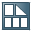#Interest Calculation### Purpose

You have the following options for calculating interest on customer and vendor accounts:

With calculation of interest on arrears

The following section describes the calculation of interest on arrears.

### Features

Interest calculation is controlled by settings made in the interest indicator. You assign an interest indicator to the master records of the customer and vendor accounts you want to calculate interest for. You make other settings for each interest calculation. These specifications take effect together with the specifications for the indicator, and sometimes have a higher priority.

Interest is calculated using the debit interest rate defined for the interest indicator in the system. Credit interest rates are used when interest is being calculated on items paid prior to their due date.

### Activities

There are different ways of calculating interest:

In either Accounts Payable or Accounts Receivable, choose Periodic Processing Interest Calculation Arrears Interest Without Open Items if you only want to calculate interest for cleared items and post the interest. The system calculates interest as of the due date for net payment.

In either Accounts Payable or Accounts Receivable, choose Periodic Processing Interest Calculation Arrears Interest With Open Items if you want to calculate interest for open and cleared items and post the interest. The system calculates interest as of the upper limit date of the last interest run.

In either Accounts Payable or Accounts Receivable, choose Periodic Processing Interest Calculation Arrears Interest Without Postings if you want to calculate interest for open and/or cleared items but do not want to post the interest. The system calculates interest as of the due date for net payment.

In each of the above cases, either the relevant fields have default entries or only the selection criteria required are displayed.

In either Accounts Payable or Accounts Receivable, choose Periodic Processing Interest Calculation Arrears Interest Custom Selections if you want to make a selection that differs from the options mentioned above.

For more information about calculating interest on arrears, see the report documentation:

RFDUZI00   Calculation of Interest on Arrears (Customers)

RFKUZI00   Calculation of Interest on Arrears (Vendors)